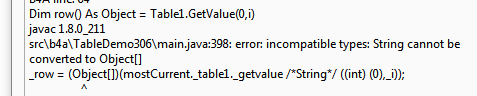# Android Question[Solved]Delete table row based on value of 1st column

#### tcgoh

##### Active Member
Hi,

I have a table with empty cell in 1st column, how do i delete the row based on the 1st column,

For i = 0 To Table1.NumberOfRows -1
Dim row() As Object = Table1.GetValue(0,i)
Dim name As String = row(0)
If name = "" Then
Table1.RemoveRow(i)
Return
End If
Next

The above don't work. Any help thanks.

#### Computersmith64

##### Well-Known Member
Is the string really empty? If so you could try:
B4X:
``````For i = 0 To Table1.NumberOfRows -1
Dim row() As Object = Table1.GetValue(0,i)
Dim name As String = row(0)
If name.Length = 0 Then
Table1.RemoveRow(i)
Return
End If
Next

'Or if there could be spaces in it

For i = 0 To Table1.NumberOfRows -1
Dim row() As Object = Table1.GetValue(0,i)
Dim name As String = row(0)
If name.Trim.Length = 0 Then
Table1.RemoveRow(i)
Return
End If
Next``````

- Colin.

#### tcgoh

##### Active Member
The line
Dim row() as object = table1.getvalue(i)
Don't match as one is object vs strings ??

#### DonManfred

##### Expert
Don't match as one is object vs strings ??
do you get any error using it?

#### tcgoh

##### Active Member
yes,#### klaus

##### Expert
I would do it like this, removing all rows with an empty value in the first column:
B4X:
``````For i = Table1.NumberOfRows -1 To 0
Dim name As String = Table1.GetValue(0, i)
If name = "" Then
Table1.RemoveRow(i)
End If
Next``````
Table1.GetValue(col, row) returns a String and not an array of objects !
Table1.GetValues(row) returns an array of Strings containing the values of all columns in the given row.

#### tcgoh

##### Active Member
I would do it like this, removing all rows with an empty value in the first column:
B4X:
``````For i = Table1.NumberOfRows -1 To 0
Dim name As String = Table1.GetValue(0, i)
If name = "" Then
Table1.RemoveRow(i)
End If
Next``````
Table1.GetValue(col, row) returns a String and not an array of objects !
Table1.GetValues(row) returns an array of Strings containing the values of all columns in the given row.
Thanks Klaus,
But this code doesn't work also. No error generated and the rows are not deleted.

#### klaus

##### Expert
Sorry, it should be:

B4X:
``````For i = Table1.NumberOfRows - 1 To 0 Step -1
Dim name As String = Table1.GetValue(0, i)
If name = "" Then
Table1.RemoveRow(i)
End If
Next``````
This code works, I tested it.

Last edited:

#### tcgoh

##### Active Member
Finally get a chance to test it. It works great Thanks Klaus.

Replies
119
Views
7K
Replies
2
Views
440
Replies
1
Views
259
Replies
1
Views
184
Replies
1
Views
753